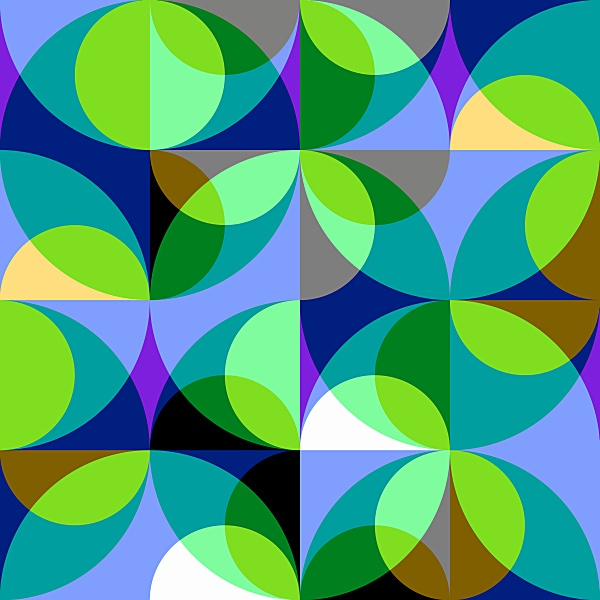Categorical logic is a branch of mathematical logic that uses category theory as its principal mathematical tool and as mathematical foundation. This  mathematical setting profoundly changes the conception of logic put forward by Frege and Russell in the beginning of 20th century both in its technical and philosophical aspects. On the technical side categorical logic inherits features from earlier constructive and algorithmic approaches to logic, in particular from realizability, lambda-calculus, intuitionistic logic and type theory. (In fact a typed intuitionistic logical calculus in the categorical setting appears to be the most natural system of logic while classical logic turns out to be a very special case that requires strong additional conditions.). This is one of the reasons why categorical logic is so successfully used in computer science. On its philosophical side categorical logic suggests a new notion of intrinsic logic that is analogous to the notion of  intrinsic geometry that made a revolution in this mathematical discipline in 19th century. Frege and Russell after Aristotle conceived of logic as a system of universal rules of reasoning independent of any particular subject domain. Categorical logic not only diversifies the notion of logic by giving a space for different systems of logic, but also provides a mechanism of adjustment of a system of logic (i.e. a formal language) to a given domain of study and thought.

Some of the research in categorical logic  sees a great dichotomy between "categorical proof theory" and "categorical model theory". Categorical proof theory is able to model different proofs of a given theorem, and compares these different proofs, using categorical concepts. Categorical model theory is an extension of traditional model theory, where models are categories. We see this meeting as encompassing both aspects of categorical logic.

Topics that  fit this Special Session include, but are not limited to, the following:

• Relationships between logic and geometry in a topos-theoretic setting
• Categorical logic and Categorical foundations of mathematics
• Sketch theory; diagrammatic syntax
• Functorial semantics and Categorical Model theory
• Quantum logic categorically
• Extensions of categorical semantics to different kinds of logics, such as modal and substructural logics
• Comparison of different categorical frameworks
Categorical Logic

Special Session

Organized by

Valeria de Paiva (Cuil. Inc, USA)

and

Andrei Rodin (University of Paris 7, France)

Invited speaker

Maria Emilia Maietti, University of Padova, Italy, "The role of the quotient completion for the foundations of constructive mathematics"

Accepted contributed talks

1.Olivia Caramello, Scuola Normale Superiore, Pisa, Italy, "Fraïssé's construction from a topos-theoretic perspective"

2.Dominique Duval, Université de Grenoble, France, "Deduction rules are fractions"

3.Luis Estrada-González, State University of Morelos, Mexico, "The other topos theory"

4.René Guitart, University Paris-Diderot 7, France, "The link between logic and geometry in the mathematical pulsation between 3-ary and 2-ary laws"

5.Michael Lieberman, University of Pennsylvania, USA, "Accessible Categories and Abstract Elementary Classes"

6. Eduardo Ochs, PURO/UFF, Brazil, "Downcasing Types"

7. Alain Prouté, University Paris-Diderot 7, France, "On the link between topoi and the vernacular of mathematics "

8. Eike Ritter and Valeria de Paiva, University of Birmingham, UK and Cuil, Inc. USA, "A fibrational semantics for System K "

9.Jean-Jacques Szczeciniarz, University Paris-Diderot 7, France, "Categories and diagrammatic proofs "

10.Vladimir L. Vasyukov, Institute of Philosophy RAS, Moscow, Russia, "Quantum Logics and Categories: Localism vs. Globalism "Sudoku 4B by Kerry Mitchell